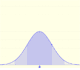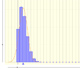Normal distribution: (Cumulative) Distribution Function

The (cumulative) distribution function of a random variable X, evaluated at x, is the probability that X will take a value less than or equal to x.In the case of a continuous distribution (like the normal distribution) it is the area under the probability density function (the 'bell curve') from the negative left (minus infinity) to x. The shaded area of the curve represents the probability that X is less or equal than x.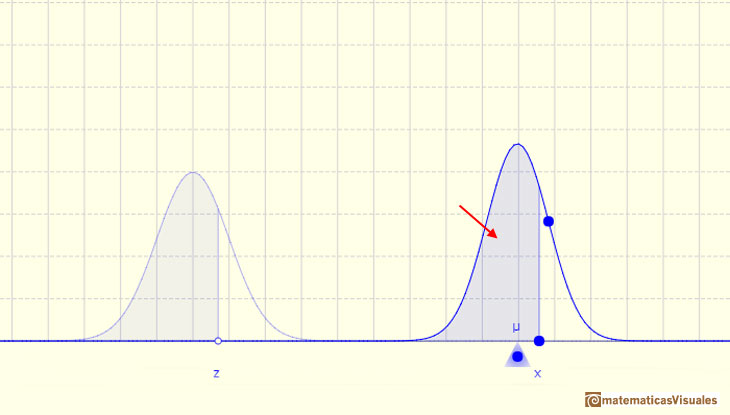The (cumulative) ditribution function F is strictly increasing and continuous. It has an S-shape.We can use the integral notation, then the (cumulative) distribution function can be written as an integral of its probability density function:In the case of the normal distribution this integral does not exist in a simple closed formula. It is computed numerically.

The Standard normal distribution plays an important roleIn some books they use a special notation for the (cumulative) distribution function in this special case of a standard normal distribution:We already know that there is a relation between any normal distribution X and the standard normal distribution Z with mean 0 and standard deviation 1-One important property of normal distributions is that if we consider intervals centered on the mean and a certain extent proportional to the standard deviation, the probability of these intervals is constant regardless of the mean and standard deviation of the normal distribution considered.

This result is much more general: Any arbitrary normal distribution X can be converted to a standard normal distribution Z by changing variables to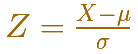You simply let the mean and variance of your random variable be 0 and 1, respectively.

This is called standardizing the normal distribution. A value from any normal distribution can be transformed into its corresponding value on a standard normal distribution. You can standardize your value by subtracting the mean and dividing the result by the standard deviation (z-score).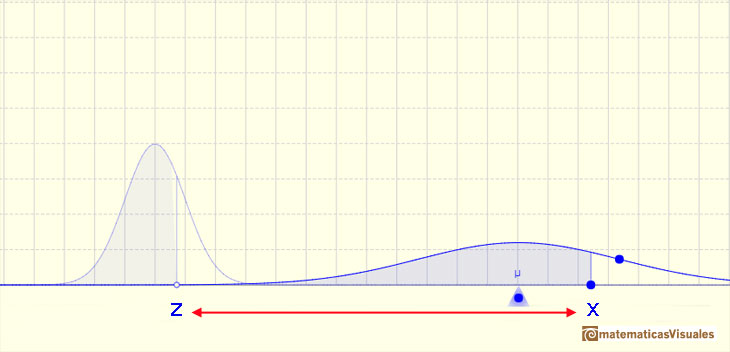There is a practical consequence of that. We know that normal distributions are a family of infinite distributions and that to calculate probabilities we need to calculate integrals that we can do only numerically. But to do these calculations we only need one table of data or only one main function in any programing language (if we are using a computer). We need only to know the integral for the standard normal distribution.

We can calculate the probability of any interval:

The probability that the random variable falls within a given range is the area under the density function for that range. We use the (cumulative) probability function to do that.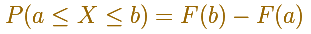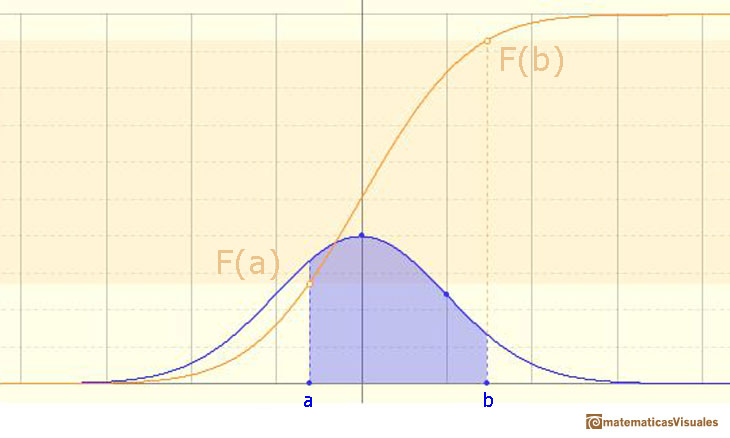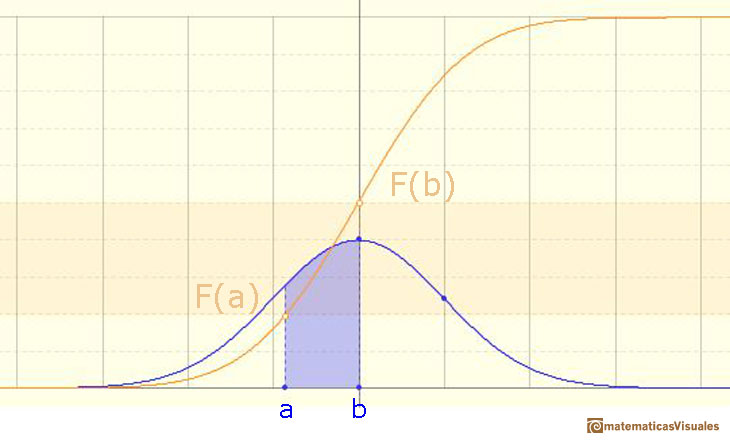We can calculate more probabilities, for example: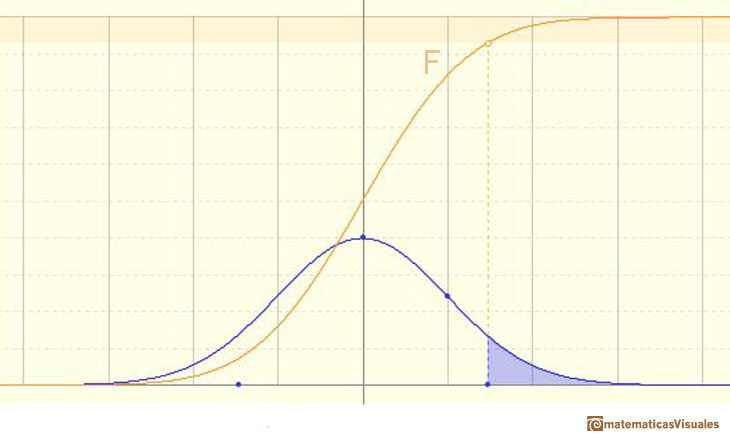An we can write: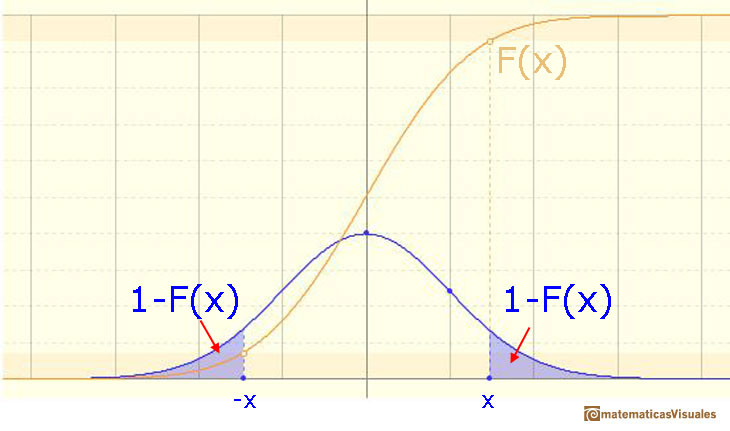We can modifying the parameters of the normal distribution.

The mean is represented by a triangle that can be seen as an equilibrium point. By dragging it we can modify the mean.

Dragging the point on the curve (which is one of the two inflexion points of the curve) we modify the standard deviation.

We can see the cumulative distribution function and how it change by modifiyng the mean (simple translation) and the standard deviation (reflecting greater or lesser dispersion of the variable).

The red dots control the vertical and horizontal scales of the graphic.

REFERENCES

George Marsaglia's article Evaluating the Normal Distribution.
Saul Stahl's article The Evolution of the Normal Distribution (Mathematics Magazine, vol. 79, (2006), pp. 96-113. From Mathematical Association of America).